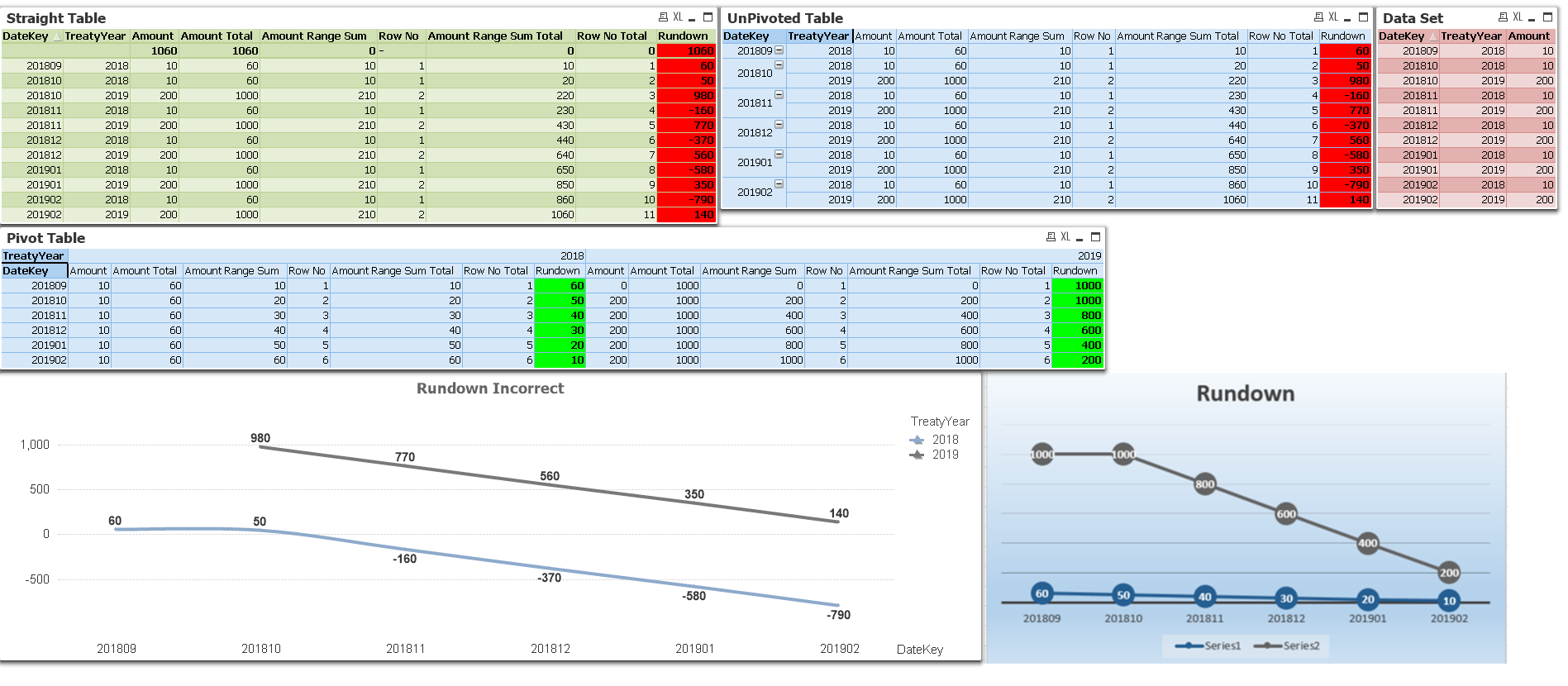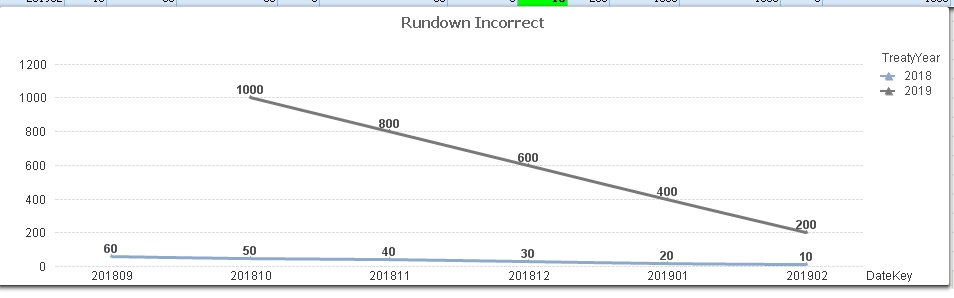# QlikView App Development

Discussion Board for collaboration related to QlikView App Development.

Highlighted
New Contributor

## Rundown Line Chart - Accumulation Issue - RangeSum

Hi,

I currently have an issue with the Range Sum / Accumulation in QV when trying to use a line chart. I have attached an example to demonstrate. I am not sure how to get the accumulation to work as required.

In my Example, please note the following objects (Screenshot Below)

• Table Box - Data Set - (Red) - Inline data loaded in script for test
• Straight Table - (Green) - The line chart is following the straight tables logic on doing the rundown, which is incorrect. This has been highlighted in red
• Un-Pivoted Table - (Blue) - This is to illustrate why the straight table is an issue and why the accumulation or range sum only works as needed when this table is pivoted. Result also highlighted in red
• Pivot Table - (Blue) - This illustrates the outcome I need in table form and is correct. Result is highlighted in Green. But I need this to be applied to a graphic such as a line chart
• Rundown Incorrect - (Line Chart) - This is the graphical version of what i need in the end, but unfortunately applies the straight table logic, which is incorrect
• Rundown - (Text Object) - This is the end expected resultRegards

Tyron

1 Solution

Accepted SolutionsMVP

## Re: Rundown Line Chart - Accumulation Issue - RangeSum

Try this

RangeSum(Sum(TOTAL <TreatyYear> Amount), -Aggr(RangeSum(Above(Sum(Amount), 1, RowNo())), TreatyYear, DateKey))2 RepliesMVP

## Re: Rundown Line Chart - Accumulation Issue - RangeSum

Try this

RangeSum(Sum(TOTAL <TreatyYear> Amount), -Aggr(RangeSum(Above(Sum(Amount), 1, RowNo())), TreatyYear, DateKey))New Contributor

## Re: Rundown Line Chart - Accumulation Issue - RangeSum

Thank you very much for your assistance Sunny.

The only issue I found is if you have any logic on your dimensions such as using as Left or Date# etc. and even add this to the expression, it breaks. But its a easy fix by just moving the formatting to script 🙂